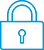cancel
Showing results for
Search instead for
Did you mean:It has been a while since anyone has replied. Simply ask a new question if you would like to start the discussion again.New member
4 2 0 1
Message 1 of 4
1,276
Flag Post

# HP Prime equation solving program returns no answer when variable has a decimal exponentHP Recommended
HP Prime Graphing Calculator

Hi, Community

I am busy trying to write a program that enables the user to select which variable is the unknown, and enter values for the other variables, after which the calculator solves for the unknown. However, whenever the variable I'm trying to solve for has an exponent that is not a whole number, the calculator returns an answer of '{}'.

Can somebody see the problem?

Code below:

EXPORT Q_MANNINGS()

BEGIN

MSGBOX(" Q = (1/N)*(A^(5/3))*(S^(1/2))/(P^(2/3)) ");

CHOOSE(Z,"SELECT UNKNOWN","Q","N","A","S","P");

IF Z==1 THEN

INPUT({N,A,S,P});

RETURN CAS.fsolve(((A^(5/3))*(S^(1/2)))/((N)*(P^(2/3)))-X,X);

END;

IF Z==2 THEN

INPUT({Q,A,S,P});

RETURN CAS.fsolve(((A^(5/3))*(S^(1/2)))/((X)*(P^(2/3)))-Q,X);

END;

IF Z==3 THEN

INPUT({Q,N,S,P});

RETURN CAS.fsolve(((X^(5/3))*(S^(1/2)))/((N)*(P^(2/3)))-Q,X);

END;

IF Z==4 THEN

INPUT({Q,N,A,P});

RETURN CAS.fsolve(((A^(5/3))*(X^(1/2)))/((N)*(P^(2/3)))-Q,X);

END;

IF Z==5 THEN

INPUT({Q,N,A,S});

RETURN CAS.fsolve(((A^(5/3))*(S^(1/2)))/((N)*(X^(2/3)))-Q,X);

END;

END;

Tags (3)
3 REPLIES 3Level 6
305 297 32 76
Message 2 of 4
Flag PostHP Recommended

fsolve is a CAS command so the variable you want to solve for, which in this case is: x, has to be in lowercase.

Besides the argument of CAS.fsolve has to be between quotes.

So I think that instead of

CAS.fsolve(((X^(5/3))*(S^(1/2)))/((N)*(P^(2/3)))-Q,X)

you have to write:

CAS.fsolve("((x^(5/3))*(S^(1/2)))/((N)*(P^(2/3)))-Q,x")New member
4 2 0 1
Message 3 of 4
Flag PostHP Recommended

Thank you very much, you helped me out a lot!Level 6
305 297 32 76
Message 4 of 4
Flag PostHP Recommended

I consider this a very helpful article concerning CAS commands in non - CAS programs:

http://www.hpmuseum.org/forum/thread-3149.html

† The opinions expressed above are the personal opinions of the authors, not of HP. By using this site, you accept the Terms of Use and Rules of Participation